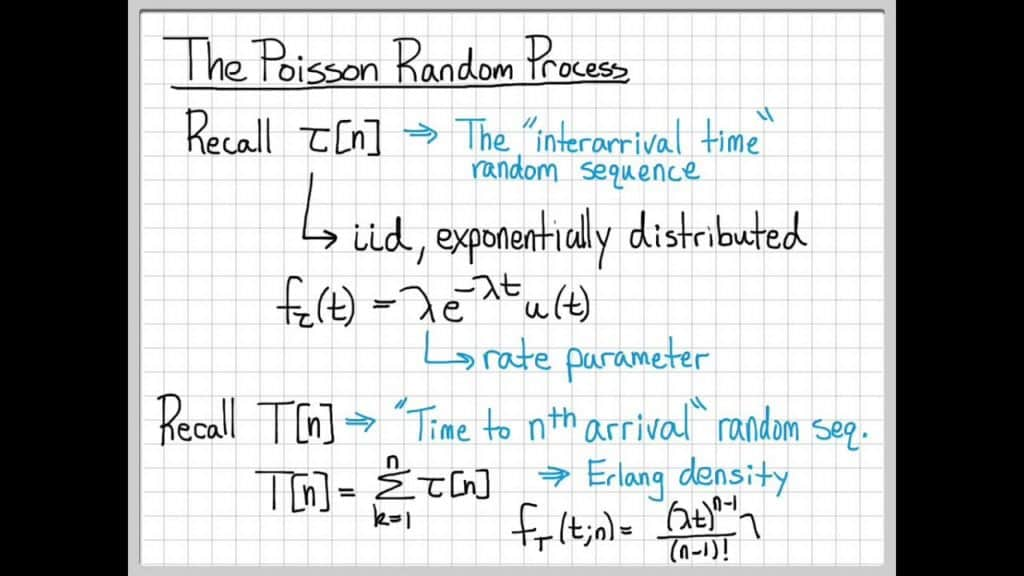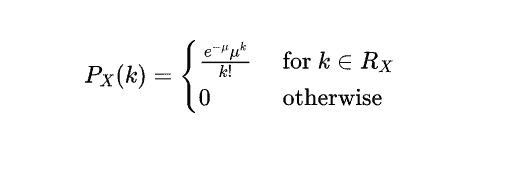poisson process代写

Definition.

Poisson random variable: Here, we briefly review some properties of the Poisson random variable that we have discussed in the previous chapters. Remember that a discrete random variable $X$ is said to be a Poisson random variable with parameter $\mu$, shown as $X \sim \operatorname{Poisson}(\mu)$, if its range is $R_{X}={0,1,2,3, \ldots}$, and its PMF is given byPMF of Poisson random variable

Here are some useful facts that we have seen before:

1. If $X \sim$ Poisson $(\mu)$, then $E X=\mu$, and $\operatorname{Var}(X)=\mu .$
2. If $X_{i} \sim$ Poisson $\left(\mu_{i}\right)$, for $i=1,2, \cdots, n$, and the $X_{i}$ ‘s are independent, then
$$X_{1}+X_{2}+\cdots+X_{n} \sim \operatorname{Poisson}\left(\mu_{1}+\mu_{2}+\cdots+\mu_{n}\right)$$
3. The Poisson distribution can be viewed as the limit of binomial distribution.
Theorem 1.

$N(t)$ has the Poisson distribution with parameter $\lambda t ;$ that is to say,
$$\mathbb{P}(N(t)=j)=\frac{(\lambda t)^{j}}{j !} e^{-\lambda t}, \quad j=0,1,2, \ldots .$$

Proof .

Condition $N(t+h)$ on $N(t)$ to obtain
\begin{aligned} \mathbb{P}(N(t+h)=j) &=\sum_{i} \mathbb{P}(N(t)=i) \mathbb{P}(N(t+h)=j \mid N(t)=i) \ &=\sum_{i} \mathbb{P}(N(t)=i) \mathbb{P}((j-i) \text { arrivals in }(t, t+h]) \ &=\mathbb{P}(N(t)=j-1) \mathbb{P}(\text { one arrival })+\mathbb{P}(N(t)=j) \mathbb{P}(\text { no arrivals })+o(h) . \end{aligned}
Thus $p_{j}(t)=\mathbb{P}(N(t)=j)$ satisfies
$$p_{j}(t+h)=\lambda h p_{j-1}(t)+(1-\lambda h) p_{j}(t)+o(h) \quad \text { if } \quad j \neq 0$$
$p_{0}(t+h)=(1-\lambda h) p_{0}(t)+o(h)$
Subtract $p_{j}(t)$ from each side of the first of these equations, divide by $h$, and let $h \downarrow 0$ to obtain
$$p_{j}^{\prime}(t)=\lambda p_{j-1}(t)-\lambda p_{j}(t) \quad \text { if } \quad j \neq 0$$
likewise
$$p_{0}^{\prime}(t)=-\lambda p_{0}(t)$$
The boundary condition is
$$p_{j}(0)=\delta_{j 0}=\left{\begin{array}{ll} 1 & \text { if } j=0 \ 0 & \text { if } j \neq 0 \end{array}\right.$$

Equations (3) and (4) form a collection of differential-difference equations for the $p_{j}(t)$. Here are two methods of solution, both of which have applications elsewhere.

Method A. Induction. Solve (4) subject to the condition $p_{0}(0)=1$ to obtain $p_{0}(t)=e^{-\lambda t}$. Substitute this into (3) with $j=1$ to obtain $p_{1}(t)=\lambda t e^{-\lambda t}$ and iterate, to obtain by induction that
$$p_{j}(t)=\frac{(\lambda t)^{j}}{j !} e^{-\lambda t}$$
Method B. Generating functions. Define the generating function
$$G(s, t)=\sum_{j=0}^{\infty} p_{j}(t) s^{j}=\mathbb{E}\left(s^{N(t)}\right)$$
Multiply (3) by $s^{j}$ and sum over $j$ to obtain
$$\frac{\partial G}{\partial t}=\lambda(s-1) G$$
with the boundary condition $G(s, 0)=1$. The solution is, as required,
(6)
$$G(s, t)=e^{\lambda(s-1) t}=e^{-\lambda t} \sum_{j=0}^{\infty} \frac{(\lambda t)^{j}}{j !} s^{j} .$$

## Second Definition of the Poisson Process:

Let $N(t)$ be a Poisson process with rate $\lambda$. Consider a very short interval of length $\Delta$. Then, the number of arrivals in this interval has the same distribution as $N(\Delta) .$ In particular, we can write
\begin{aligned} P(N(\Delta)=0) &=e^{-\lambda \Delta} \ &=1-\lambda \Delta+\frac{\lambda^{2}}{2} \Delta^{2}-\cdots \text { (Taylor Series) } \end{aligned}
Note that if $\Delta$ is small, the terms that include second or higher powers of $\Delta$ are negligible compared to $\Delta$. We write this as
$$P(N(\Delta)=0)=1-\lambda \Delta+o(\Delta)$$
Here $o(\Delta)$ shows a function that is negligible compared to $\Delta$, as $\Delta \rightarrow 0$. More precisely, $g(\Delta)=o(\Delta)$ means that
$$\lim _{\Delta \rightarrow 0} \frac{g(\Delta)}{\Delta}=0$$
Now, let us look at the probability of having one arrival in an interval of length $\Delta$.
\begin{aligned} P(N(\Delta)=1) &=e^{-\lambda \Delta} \lambda \Delta \ &=\lambda \Delta\left(1-\lambda \Delta+\frac{\lambda^{2}}{2} \Delta^{2}-\cdots\right) \text { (Taylor Series) } \ &=\lambda \Delta+\left(-\lambda^{2} \Delta^{2}+\frac{\lambda^{3}}{2} \Delta^{3} \cdots\right) \ &=\lambda \Delta+o(\Delta) . \end{aligned}
We conclude that
$$P(N(\Delta)=1)=\lambda \Delta+o(\Delta)$$
Similarly, we can show that
$$P(N(\Delta) \geq 2)=o(\Delta)$$
In fact, equations $11,1,11,2$, and $11.3$ give us another way to define a Poisson process.

## Sum of two possion process

You might sometimes want to add together two (or more) independent Poisson processes, where by independent I mean that any two increments, one from each process, are independent random variables. For example, $N_{1}$ might be counting the number of retirements from Company #1, which occur at the Poisson rate 10 per year, and $N_{2}$ might be counting those from Company #2, which occur at the Poisson rate 15 per year; for some reason you are interested in the total retirements from the two companies combined. It seems plausible that the combined retirements occur at the rate $10+15=25$ per year. What is not at all obvious, however, nor simple to prove, is that 25 is a Poisson rate – that is, that the combined number of retirements constitutes a Poisson process with rate 25 per year. Since the proof of this result is beyond the scope of this note, I’ll simply state the result.

Theorem 2.

Fact (sum of Poisson processes) Suppose that the Poisson processes $N_{1}$ with rate function $\lambda_{1}$ and $N_{2}$ with rate function $\lambda_{2}$ are independent. Then the counting process $N$ defined by $N(t)=$ $N_{1}(t)+N_{2}(t)$ is a Poisson process with rate function $\lambda$ given by $\lambda(t)=\lambda_{1}(t)+\lambda_{2}(t)$.

New stochastic processes can be created as a mixture of Poisson processes. Since mixture distributions are important in other contexts as well and may not be familiar to you, please allow me to ramble a bit about mixtures in general. It might help to have a simple example first.

Example 1.16 Imagine a box full of fair dice; $20 \%$ of the dice are four-sided with the faces numbered one through four, and $80 \%$ are six-sided with the faces numbered one through six. If you repeatedly
$$9$$
roll a four-sided die, you’ll be seeing values from a Uniform random variable on the integers $1,2,3$, 4, all equally likely. And if you repeatedly roll a six-sided die, you’ll be seeing values from a Uniform random variable on the integers $1,2,3,4,5,6$, all equally likely.

But suppose that you reach into the box, grab a die at random (so a $20 \%$ chance of grabbing a four-sided die), roll it once, record the number, and return the die to the box. And then you repeat that process over and over, grabbing and rolling a random die each time. The results you will see are not from a Uniform random variable on $1,2,3,4$, nor from a Uniform random variable on 1,2, $3,4,5,6$. Rather, they are from a $20 / 80$ mixture of those two distributions.

Suppose that you want to compute the probability of rolling a 3 with a randomly chosen die – that is, $\operatorname{Pr}[N=3]$ where $N$ follows the mixture distribution. The rigorous mathematical computation of this proceeds as follows:
\begin{aligned} \operatorname{Pr}[N=3] &=\operatorname{Pr}[N=3 \text { and four-sided }]+\operatorname{Pr}[N=3 \text { and six-sided }] \ &=\operatorname{Pr}[\text { four-sided }] \operatorname{Pr}[N=3 \mid \text { four-sided }]+\operatorname{Pr}[\text { six-sided }] \operatorname{Pr}[N=3 \mid \text { six-sided }] \ &=(0.2)\left(\frac{1}{4}\right)+(0.8)\left(\frac{1}{6}\right)=\frac{11}{60}=0.18333 . \end{aligned}
The more intuitive Mixing Method approach is as follows: pretend you know which case you are in and compute the answers $[1 / 4$ and $1 / 6$ in this case $]$, and then compute the expected value of those answers as the cases vary [so $(0.2)(1 / 4)+(0.8)(1 / 6)=11 / 60$ as above]. Let’s use this approach to compute the expected value of $N .$ If you knew you had a four-sided die, the expected value would be $2.5$, while it would be $3.5$ for a six-sided die. So what is the expected value for a random die? Just the expected value of those two answers as the die-type varies: $(0.2)(2.5)+(0.8)(3.5)=3.3 .$ \Phi
For the Mixing Method used in Example $1.16$ to be valid, the quantity you are computing for the mixture distribution must involve only a linear computation with the probability function (or density function for a continuous-type random variable) of the mixture – that is, no squaring of probabilities, no dividing by probabilities, et cetera. This is certainly true for an unconditional probability for the mixture, as just illustrated with $\operatorname{Pr}[N=3]$ above. It’s also valid for the expected value, which is just a sum of probabilities times values of the variable. Likewise for, say, the second moment, since that is just a sum of probabilities times squared values of the variable; in Example 1.16, for example, $\mathrm{E}\left[N^{2}\right]=(0.2)(30 / 4)+(0.8)(91 / 6)=409 / 30=13.6333 .$ But not so for the variance, since the variance involves the square of the mean and thus the squaring of probabilities. In Example $1.16$, for instance, $\operatorname{Var}[N]$ is correctly computed as the mixture’s second moment $409 / 30$ minus the square of the mixture’s mean 3.3: $\operatorname{Var}[N]=409 / 30-3.3^{2}=2.7433$. Mixing the two variances $15 / 12$ and $35 / 12$ in the $20 / 80$ proportion gives the incorrect value $2.5833 .$泊松过程代写认准UpriviateTA